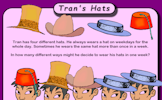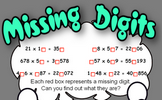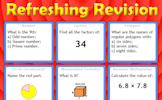# Probability

### Term 3 starting in week 7 :: Estimated time: 2 weeks

• Single event probability (review)
• Relative frequency - include convergence
• Expected outcomes
• Independent events
• Use diagrams to work out probabilities

For higher-attaining pupils:

• Use tree diagrams
• Use tree diagrams to solve 'without replacement' problems

This page should remember your ticks from one visit to the next for a period of time. It does this by using Local Storage so the information is saved only on the computer you are working on right now.

## Lesson Starters

Here are some suggestions for whole-class, projectable resources which can be used at the beginnings of each lesson in this block.

### 1st Lesson#### Tran's Hats

In how many different ways might Tran decide to wear his hats in one week?

### 2nd LessonStand at the point between the classroom walls to represent a given number.

### 3rd Lesson#### Just Four Questions

Four mathematical questions to think about at the beginning of the lesson.

### 4th Lesson#### Windmill Sequence

Find the value of the missing term of the sequence. It is easier than you may think!

### 5th Lesson#### Missing digits

Find which digits are missing from the randomly generated calculations.

### 6th Lesson#### Refreshing Revision

It is called Refreshing Revision because every time you refresh the page you get different revision questions.

Some of the Starters above are to reinforce concepts learnt, others are to introduce new ideas while others are on unrelated topics designed for retrieval practice or and opportunity to develop problem-solving skills.

White Rose ResourcesEnd of block assessments provide a quick progress check at the end of each block of learning to make sure students have understood the content covered. This Scheme of Learning was produced by White Rose Maths and is used here with permission granted on 30th June 2021.For All: